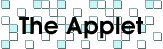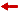So, your browser can't run Java applets? At least, it probably crashes less than mine!

### Definitions

• A geometric problem is a formal input/output problem, where the input is a set of points in the plane, and the output can be a point, a line, a circle, or a combination of these.

• A construction is a geometric algorithm that solves a particular geometric problem under our model of computation.

• A construction is said to be valid if it works for "almost all" input configurations (i.e. it will crash with probability zero if the input points are placed at random).

• A construction is said to be robust if it works for all input configurations without exception.

### Rules of the game

• The constructions are restricted to be sequential lists of operations (no loop, conditional or other form of flow control).

• The constructions are required to be valid, but not necessarily robust.

• The best valid construction for a particular problem is the one with the smallest cost.

• In case of a tie, a construction with fewer steps (hence using more subroutines) is considered preferable.

• The solution of a problem cannot be used as a subroutine to solve that same problem in one step (!).

Your applet is quite complicated. Where's the documentation?
 There's no documentation because I myself never read an applet's documentation. Read the contents of the yellow help-box, it is helpful! And experiment, I don't think that you can hurt yourself.
How does the applet check whether a given construction is valid or not?
 The program computes the construction in double precision and expects the goal to be reached within a certain distance. If there are more than 2 free points, then you need to move the input points to convince yourself that the construction works in general. Of course, this is not a rigorous proof of correctness, but it works well in practice.
How good is double precision?
 Unit roundoff is 2-53 which is approximately 10-16. The construction that you see on your screen is actually computed to 1/100 the size of a proton. Two points are considered equal if they lie within the size of an atom. :)
Since you favor the use of subroutines, aren't you afraid to get solutions that call each other, causing circular thinking?
 No. Every solution given by the applet can be recursively expanded down to lines and circles.
Why did you put 4 impossible constructions at the end of your problem list?
 So that people claiming to have solved these problems can test their purported solutions with the applet instead of bothering mathematicians.
A lot of constructions are from you. What's the deal?
 I would like some variety in the credit line too! If you can find one of my constructions in the literature of before 1998, tell it to me. I'll give full credit to the earliest reference. By the way, I've got some help from a program I've written that can search a couple thousand constructions per second. Most of the short solutions were proven to be cost-optimal by that program.
What's that pattern that you use as a background?
 This is a pattern that you can draw with compass alone, given 2 points to start with.Back to The Complexity of Geometric Constructions